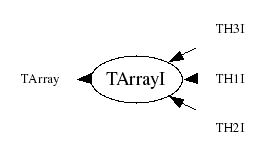library: libCore#include "TArrayI.h"

# TArrayI

class description - header file - source file

## class TArrayI: public TArray### Function Members (Methods)

Display options:
Show inherited
Show non-public
public:
 TArrayI() TArrayI(Int_t n) TArrayI(const TArrayI& array) TArrayI(Int_t n, const Int_t* array) virtual ~TArrayI() void AddAt(Int_t i, Int_t idx) void Adopt(Int_t n, Int_t* array) Int_t At(Int_t i) const static TClass* Class() void Copy(TArrayI& array) const const Int_t* GetArray() const Int_t* GetArray() Int_t TArray::GetSize() const Stat_t GetSum() const virtual TClass* IsA() const TArrayI& operator=(const TArrayI& rhs) Int_t& operator[](Int_t i) Int_t operator[](Int_t i) const static TArray* TArray::ReadArray(TBuffer& b, const TClass* clReq) void Reset() void Reset(Int_t val) virtual void Set(Int_t n) void Set(Int_t n, const Int_t* array) virtual void ShowMembers(TMemberInspector& insp, char* parent) virtual void Streamer(TBuffer& b) void StreamerNVirtual(TBuffer& b) static void TArray::WriteArray(TBuffer& b, const TArray* a)
protected:
 Bool_t TArray::BoundsOk(const char* where, Int_t at) const Bool_t TArray::OutOfBoundsError(const char* where, Int_t i) const

### Data Members

public:
 Int_t* fArray [fN] Array of fN 32 bit integers Int_t TArray::fN Number of array elements

## Class Description

```
TArrayI

Array of integers (32 bits per element).

```
TArrayI()
``` Default TArrayI ctor.
```
TArrayI(Int_t n)
``` Create TArrayI object and set array size to n integers.
```
TArrayI(Int_t n, const Int_t *array)
``` Create TArrayI object and initialize it with values of array.
```
TArrayI(const TArrayI &array)
``` Copy constructor.
```
TArrayI & operator=(const TArrayI &rhs)
``` TArrayI assignment operator.
```
~TArrayI()
``` Delete TArrayI object.
```
``` Adopt array arr into TArrayI, i.e. don't copy arr but use it directly
in TArrayI. User may not delete arr, TArrayI dtor will do it.
```
``` Add Int_t c at position i. Check for out of bounds.
```
void Set(Int_t n)
``` Set size of this array to n ints.
A new array is created, the old contents copied to the new array,
then the old array is deleted.
This function should not be called if the array was declared via Adopt.
```
void Set(Int_t n, const Int_t *array)
``` Set size of this array to n ints and set the contents.
This function should not be called if the array was declared via Adopt.
```
void Streamer(TBuffer &b)
``` Stream a TArrayI object.
```
Int_t At(Int_t i)

void Copy(TArrayI &array)
`{array.Set(fN,fArray);}`
const Int_t * GetArray()
`{ return fArray; }`

`{ return fArray; }`

`{Stat_t sum=0; for (Int_t i=0;i<fN;i++) sum+=fArray[i]; return sum;}`
void Reset()
`{memset(fArray, 0, fN*sizeof(Int_t));}`
void Reset(Int_t val)
`{for (Int_t i=0;i<fN;i++) fArray[i] = val;}`

Author: Rene Brun 06/03/95
Last update: root/cont:\$Name: \$:\$Id: TArrayI.cxx,v 1.14 2005/11/16 20:07:50 pcanal Exp \$